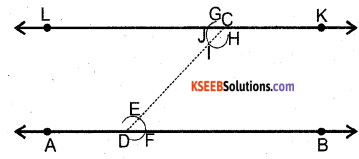# KSEEB Solutions for Class 7 Maths Chapter 10 Practical Geometry Ex 10.1

Students can Download Chapter 10 Practical Geometry Ex 10.1, Question and Answers, Notes Pdf, KSEEB Solutions for Class 7 Maths, Karnataka State Board Solutions help you to revise complete Syllabus and score more marks in your examinations.

## Karnataka State Syllabus Class 7 Maths Chapter 10 Practical Geometry Ex 10.1

Question 1.
Draw a line, say AB, take a point C out-side it. Through C, draw a line parallel to AB using ruler and compasses only.
Solution:Steps of Construction

1. Draw a line AB. Take a point C outside it. Take any point D on AB and join C to D.
2. With D as centre and a convenient radius, draw an arc cutting AB at F and CD at E.
3. Now with C as centre and the same radius as in step 2, draw an arc GH cutting CD at I.
4. Place the pointed tip of the compasses at F and adjust the opening so that the pencil tip is at E.
5. With the same opening as in step 4 and with I as centre, draw an arc cutting the are GH at J.
6. Now Join CJ to draw a line ‘KL’. KL is the required parallel line.Question 2.
Draw a line l. Draw a perpendicular to l at any point on l. On this perpendicular choose a point X, 4 cm away from l. Through X, draw a line m parallel to l.
Solution:Steps of Construction

1. Draw a line l, take any point A on line l.
2. Construct an angle of 90° at point A of line l and draw a line AL perpendicular to line l.
3. Mark a point X on AL such that AX = 4 cm
4. At X construct an angle of 90° and draw a line XC perpendicular to line AL.
5. The line XC (line m) is the required line through X such that m || l.Question 3.
Let l be a line and P be a point on l. Through P, draw a line m parallel to l. Now join P to any point Q on l. Choose any other point R on m. Through R, draw a line parallel to PQ. Let this meet l at S. What shape do the two sets of parallel lines enclose?
Solution:Steps of constructions

1. Draw a line l and take a point P, not on it. Take any point Q on l. Join Q to P
2. Draw a line m parallel to the line l, as shown in the figure. line m || line l.
3. Join P to any point Q on l.
4. Choose any point R on m & Join R to Q.
5. Through R, draw a line n parallel to the line PQ. Let the line n meet the line l at S.
6. The shape enclosed by the two sets of parallel lines is a parallelogram.
error: Content is protected !!